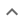•# Linear equations with one unknown

We think you wrote:

###This solution deals with linear equations with one unknown.

Solution foundx=2x=0

## Step by Step Solution## Step  2  :

#### Pulling out like terms :

Pull out like factors :

2x - 4  =   2 • (x - 2)

#### Equation at the end of step  2  :

  2x • (x - 2)  = 0


## Step  3  :

#### Theory - Roots of a product :

A product of several terms equals zero.

When a product of two or more terms equals zero, then at least one of the terms must be zero.

We shall now solve each term = 0 separately

In other words, we are going to solve as many equations as there are terms in the product

Any solution of term = 0 solves product = 0 as well.

#### Solving a Single Variable Equation :

Solve  :    2x = 0

Divide both sides of the equation by 2:
x = 0

#### Solving a Single Variable Equation :

Solve  :    x-2 = 0

Add  2  to both sides of the equation :

x = 2

1.  x = 2
2.  x = 0